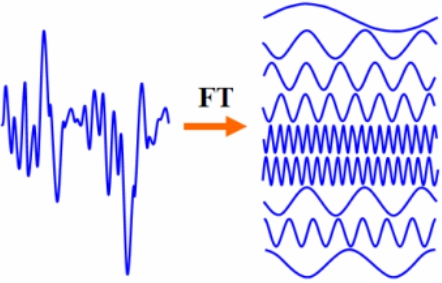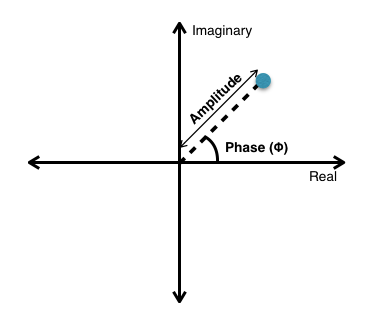# Performing Fourier Transforms in the PSLab Android App

Oscilloscope is one of the key features of PSLab. When periodic signals such as sine waves are read by the Oscilloscope, curve fitting functions are used to construct a curve that has the best fit to a series of data points. In PSLab, the sine curve fitting involves the Fourier Transforms. FFT (short for “Fast Fourier Transform”) is nothing more than a curve-fit of sines and cosines to some given data. In order to understand the implementation of Fourier Transforms in PSLab Android App let’s first have a look at the Fourier transform equations.The first equation here is the Forward Fourier transform. It converts the function of time (t) into the function of frequency (ω).
The second equation is Inverse Fourier transform. It does the opposite to first equation ie. it converts the function of frequency (ω) into the function of time (t).

So, first I will answer what is transform?
It is the mapping between two different sets of domains. In this case, the information is changed from the time domain to frequency domain. The data in these domains look different but represent the same information. A transform will get you from one representation to another.

Fourier transforms converts between the time domain f(t) and the frequency domain F(ω).
Performing Fourier Transforms in Android
Let’s perform Forward Fourier transform. This means it converts the function of time (t) into the function of frequency (ω). We will use Apache Maths Commons to perform Fourier transforms. Since we have finite input data set we will calculate Discrete Fourier Transform (DFT).
The algorithm which is being used here is Fast Fourier Transform (FFT) which is the best algorithm to calculate Fourier transforms.

```FastFourierTransformer fastFourierTransformer =
new FastFourierTransformer(DftNormalization.STANDARD);
```

Here we are creating an instance of FastFourierTransformer which passed STANDARD normalization convention to its constructor. Normalization other than STANDARD is UNITARY.
Complex complex[] = fastFourierTransformer.transform(input, TransformType.FORWARD);

Here, input array and TransformType. FORWARD is also passed to transform method. Input is an array of data representing time whereas TransformType. FORWARD defines the type of Fourier transform that should be performed ie. forward or inverse.

```Complex complex[] = fastFourierTransformer.transform(input, TransformType.FORWARD);
```

The output will be an array of complex number. Each data point will be represented like the following graph in the complex plane.Suppose the amplitude of the data point given in above graph is 1 and phase shift is 45°. So, solving this we will get 2/√2 as both real and imaginary components. Therefore, F (ω) would be 1/√2 + (1/√2) i.
Dealing with Complex numbers in Java
A complex number has both real and imaginary part. Using Apache Maths Commons we can use Complex to represent a complex number.

```Complex number = new Complex(1,2);
System.out.println(number);
```
```Output
1.0 + 2.0i```

We can also get real and imaginary parts separately of Complex numbers.

```System.out.println(number.getReal());
System.out.println(number.getImaginary());
```
```Output
1.0
2.0```

The array of the Complex numbers can be implemented like the following

```Complex[] number;
Complex c1 = new Complex(1, 2);
Complex c2 = new Complex(3, 4);
number = new Complex[]{c1, c2};
System.out.println(Arrays.toString(number));
System.out.println(number);
```
```Output

[(1.0, 2.0), (3.0, 4.0)]
(3.0, 4.0)```

### Resources

This site uses Akismet to reduce spam. Learn how your comment data is processed.